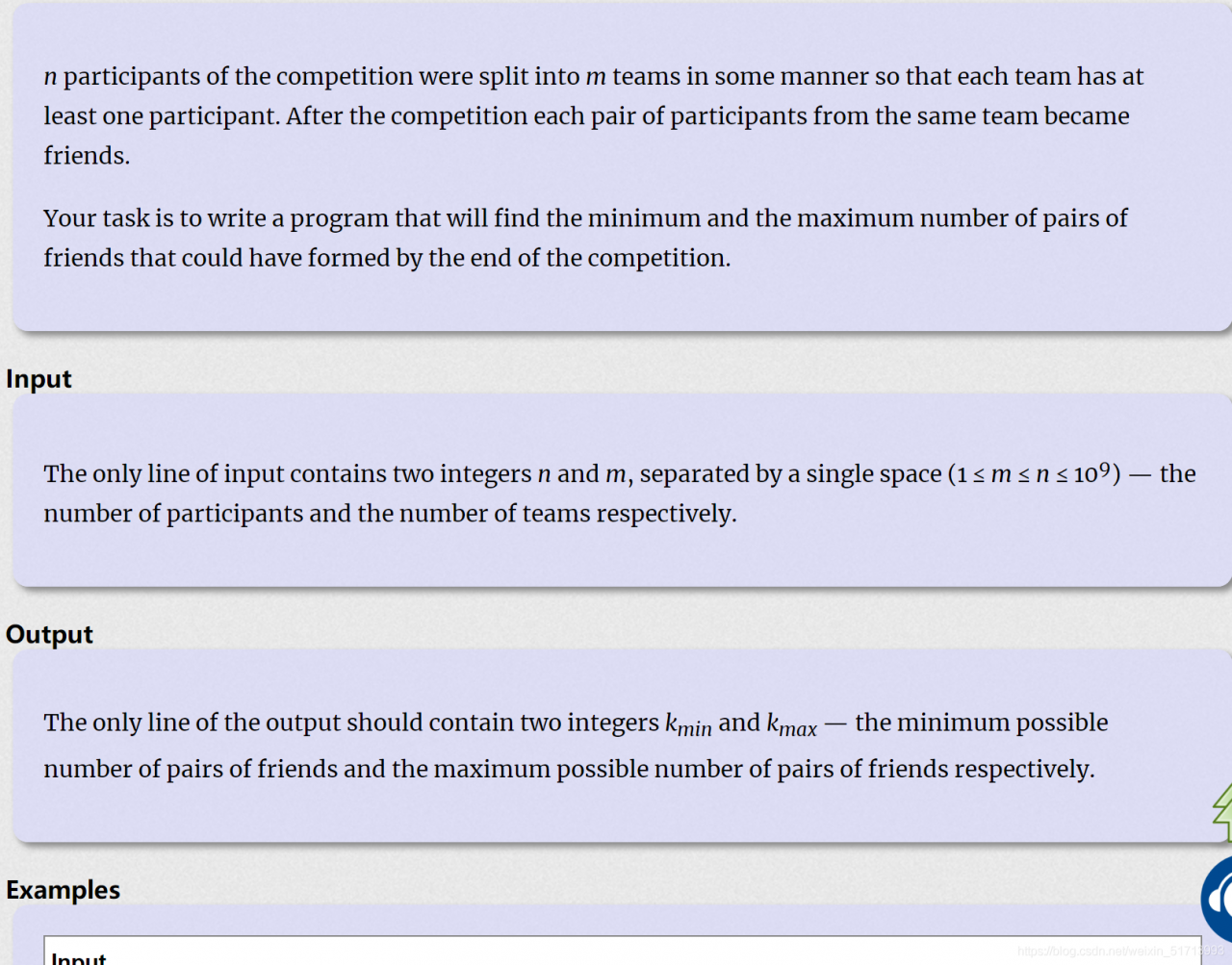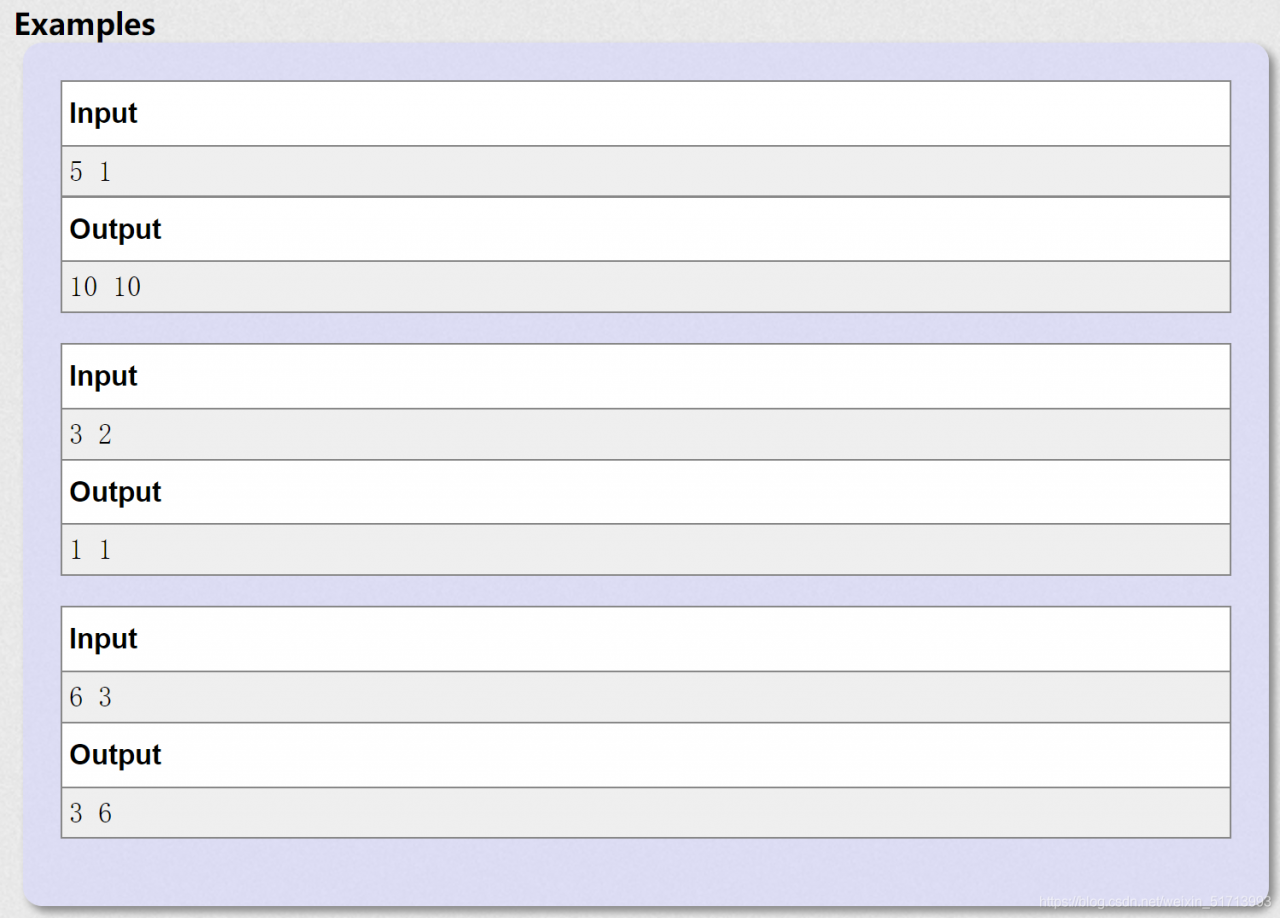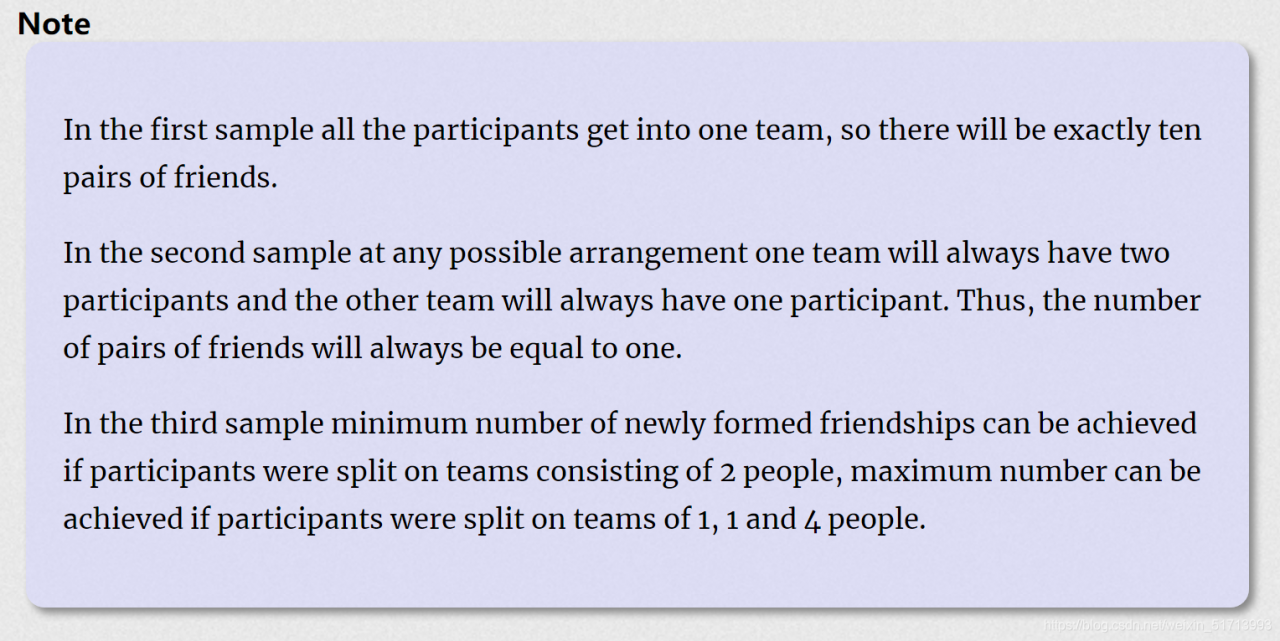# P – Random Teamsmathematical knowledge

``````#include <iostream>
#include <string.h>
#include <algorithm>

using namespace std;
int main()
{
int t;
long long m,n;
cin>>n>>m;

cout<<(m-n%m)*(n/m)*(n/m-1)/2+(n%m)*(n/m+1)*(n/m)/2<<" "<<(n-m+1)*(n-m)/2<<endl;

return 0;
}
``````